# 从TensorRT看INT8量化原理

## Low Precision Inference

TensorRT 的INT8模式只支持计算能力为6.1的GPU（Compute Capability 6.1 ），比如： GP102 (Tesla P40 and NVIDIA Titan X), GP104 (Tesla P4), and GP106 GPUs，主要根源是这些GPU支持 DP4A硬件指令。DP4A下面会稍微介绍一下。

## TensorRT INT8 Inference

动态范围最小正数
FP32 3.4×1038 +3.4×1038−3.4×1038 +3.4×1038 1.4×10451.4×10−45
FP16 65504 +65504−65504 +65504 5.96×1085.96×10−8
INT8 128 +127−128 +127 11

### Quantization

$FP32 \quad Tensor (T)=scale\_factor(sf) * 8-bit Tensor(t)+FP32\_bias (b)$

$T=sf * t$

$sf$是每一层上每一个tensor的换算系数或称比例因子（scaling factor），因此现在的问题就变成了如何确定比例因子。然后最简单的方法是下图这样的: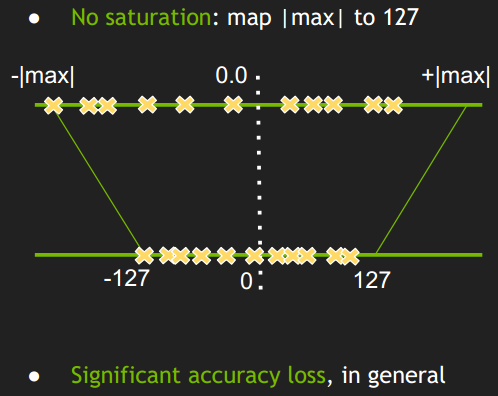• 简单的将一个tensor 中的 -|max| 和 |max| FP32 value 映射为 -127 和 127 ，中间值按照线性关系进行映射。
• 称这种映射关系为不饱和的（No saturation ），对称的。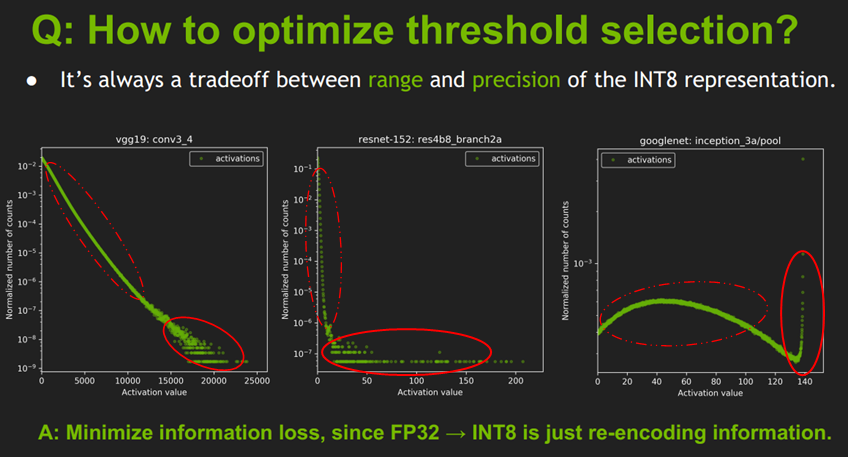• 上图是一些网络模型中间层的 激活值统计，横坐标是激活值，纵坐标是统计数量的归一化表示，这里是归一化表示，不是绝对数值统计；
• 这个激活值统计 针对的是一批图片，不同的图片输出的激活值不完全相同。所以图上并不是一条曲线而是多条曲线（一张图片对应一条曲线，或者称为散点图更好一点），只不过前面一部分重复在一块了（红色虚线圈起来的部分），说明对于不同图片生成的大部分激活值其分布是相似的；但是在激活值比较大时（红色实线圈起来的部分），曲线不重复了，一个激活值对应多个不同的统计量，这时的激活值分布就比较乱了。
• 后面这一部分在整个层中是占少数的（占比很小，比如10^-9, 10^-7, 10^-3），因此后面这一段完全可以不考虑到映射关系中去，保留激活值分布的主方向。开始我以为网络之所以能把不同类别的图片分开是由于后面实线部分的差异导致的，后来想了一下：这个并不包含空间位置的分布，只是数值上的分布，所以后面的应该对结果影响不大。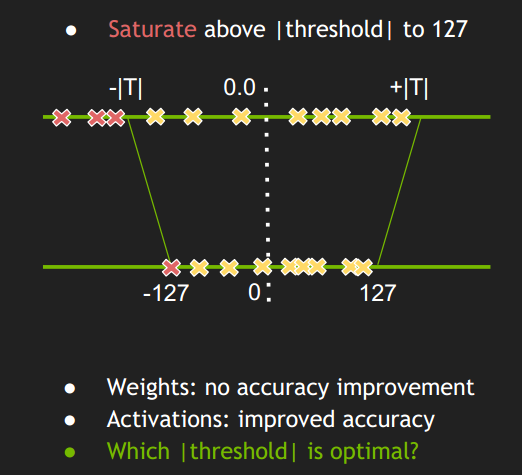• 这种做法不是将 ±|max| 映射为 ±127，而是存在一个 阈值 |T| ，将 ±|T| 映射为±127，显然这里 |T|<|max|。
• 超出 阈值 ±|T| 外的直接映射为阈值 ±127。比如上图中的三个红色点，直接映射为-127。
• 称这种映射关系为饱和的（Saturate ），不对称的。
• 只要 阈值 选取得当，就能将分布散乱的较大的激活值舍弃掉，也就有可能使精度损失不至于降低太多。

• 假设我们要给一个信息进行完美编码，那么最短平均编码长度就是信息熵。

• 如果编码方案不一定完美（由于对概率分布的估计不一定正确），这时的平均编码长度就是交叉熵。

平均编码长度 = 最短平均编码长度 + 一个增量

交叉熵在深度学习中广泛使用，衡量了测试集标签分布和模型预测分布之间的差异程度。

• 编码方法不一定完美时，平均编码长度相对于最小值的增加量（即上面那个增量）是相对熵。

$H(p)=H(X)=E_{x \sim p(x)} [-logp(x)]=- \sum_{i=1}^{n} p(x)log p(x)$

$H(p)=H(X)=E_{x \sim p(x)}[-logp(x)]=- \int p(x)log p(x)dx$

$D_{KL}(p||q)= \sum_{i=1}^{N} [p(x_{i})log p(x_{i})-p(x_{i})log q(x_{i})]= \sum_{i=1}^{N} p(x_{i})log \frac{p(x_{i})}{q(x_{i})}$

P，Q分别称为 reference_distribution、 quantize _distribution

### Calibration

Note: The calibration set must be representative of the input provided to TensorRT at runtime; for example, for image classification networks, it should not consist of images from just a small subset of categories. For ImageNet networks, around 500 calibration images is adequate.

• 首先在 校准集上 进行 FP32 inference 推理；
• 对于网络的每一层（遍历）：
• 收集这一层的激活值，并做 直方图（histograms ），分成几个组别（bins）（官方给的一个说明使用的是2048组），分组是为了下面遍历 |T| 时，减少遍历次数；
• 对于不同的 阈值 |T| 进行遍历，因为这里 |T|的取值肯定在 第128-2047 组之间，所以就选取每组的中间值进行遍历；
• 选取使得 KL_divergence(ref_distr, quant_distr) 取得最小值的 |T|。
• 返回一系列 |T|值，每一层都有一个 |T|。创建 CalibrationTable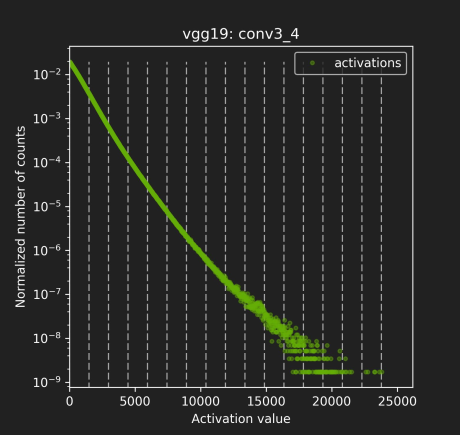校准的过程可以参考一下这个：https://www.jianshu.com/p/43318a3dc715 , 这篇文章提供了一个详细的根据KL散度来将原始信息进行编码的例子，包括直方图的使用。跟这里的校准过程极为相像。

//首先分成 2048个组，每组包含多个数值（基本都是小数）
Input: FP32 histogram H with 2048 bins: bin[ 0 ], …, bin[ 2047 ]

For i in range( 128 , 2048 ): // |T|的取值肯定在 第128-2047 组之间,取每组的中点
reference_distribution_P = [ bin[ 0 ] , ..., bin[ i-1 ] ] // 选取前 i 组构成P，i>=128
outliers_count = sum( bin[ i ] , bin[ i+1 ] , … , bin[ 2047 ] ) //边界外的组
reference_distribution_P[ i-1 ] += outliers_count //边界外的组加到边界P[i-1]上，没有直接丢掉
P /= sum(P) // 归一化

// 将前面的P（包含i个组，i>=128），映射到 0-128 上，映射后的称为Q，Q包含128个组，
// 一个整数是一组
candidate_distribution_Q = quantize [ bin[ 0 ], …, bin[ i-1 ] ] into 128 levels

//这时的P（包含i个组，i>=128）和Q向量（包含128个组）的大小是不一样的，无法直接计算二者的KL散度
//因此需要将Q扩展为 i 个组，以保证跟P大小一样
expand candidate_distribution_Q to ‘ i ’ bins

Q /= sum(Q) // 归一化
//计算P和Q的KL散度
divergence[ i ] = KL_divergence( reference_distribution_P, candidate_distribution_Q)
End For
//找出 divergence[ i ] 最小的数值，假设 divergence[m] 最小，
//那么|T|=( m + 0.5 ) * ( width of a bin )
Find index ‘m’ for which divergence[ m ] is minimal
threshold = ( m + 0.5 ) * ( width of a bin )

• 计算KL散度 KL_divergence(P, Q) 时，要求序列P和Q的长度一致，即 len(P) == len(Q)；
• Candidate_distribution_Q 是将 P 线性映射到 128个bins得到的，长度为128。而reference_distribution_P 包含 i （i>=128）个 bins （bin - bin[i-1] ），二者长度不等；
• 需要将 candidate_distribution_Q 扩展回 i 个bins 然后才能与 i个bins 的 reference_distribution_P计算KL散度。

• 假设reference_distribution_P 包含 8 个bins（这里一个bin就只包含一个数据）:

P = [ 1, 0, 2, 3, 5, 3, 1, 7]

• 我们想把它映射为 2 个bins，于是 4个一组合并：

[1 + 0 + 2 + 3 , 5 + 3 + 1 + 7] = [6, 16]

• 然后要成比例的 扩展回到 8个组，保留原来是0的组：

Q = [ 6/3, 0, 6/3, 6/3, 16/4, 16/4, 16/4, 16/4] = [ 2, 0, 2, 2, 4, 4, 4, 4]

• 然后对 P和Q进行标准化：

P /= sum(P) 、Q /= sum(Q)

• 最后计算散度：

result = KL_divergence(P, Q)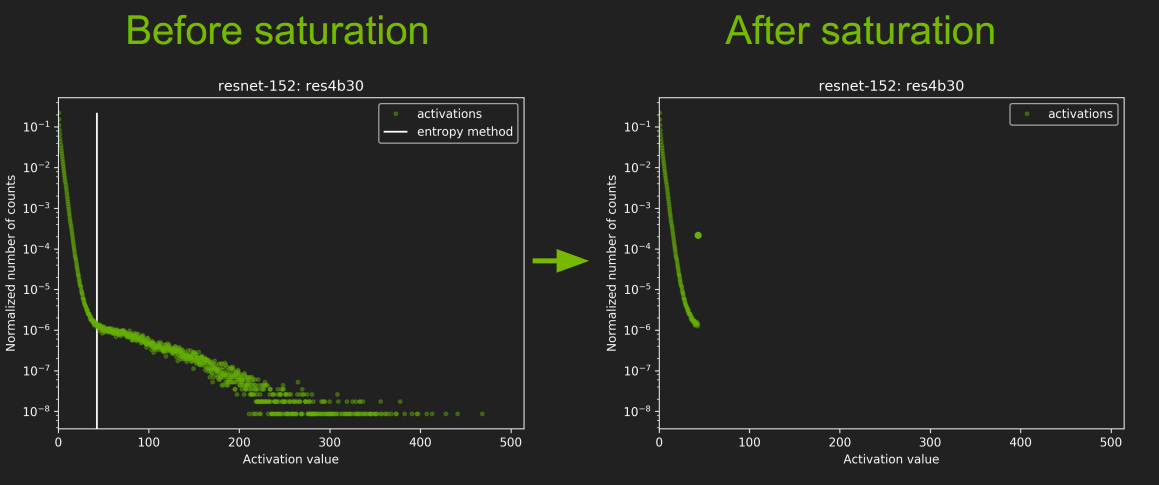• 图中那个白线就是 |T|的取值，不过怎么还小于128了，有点没搞明白。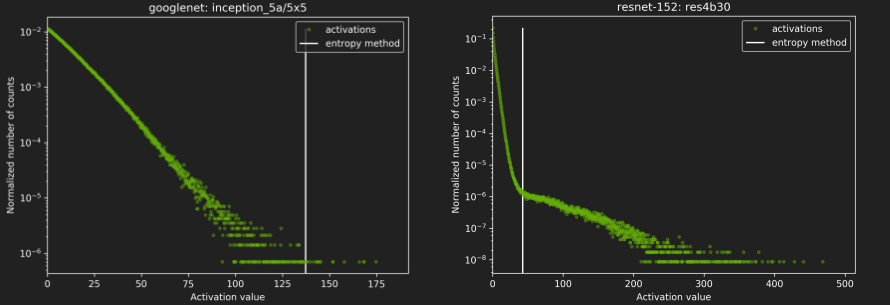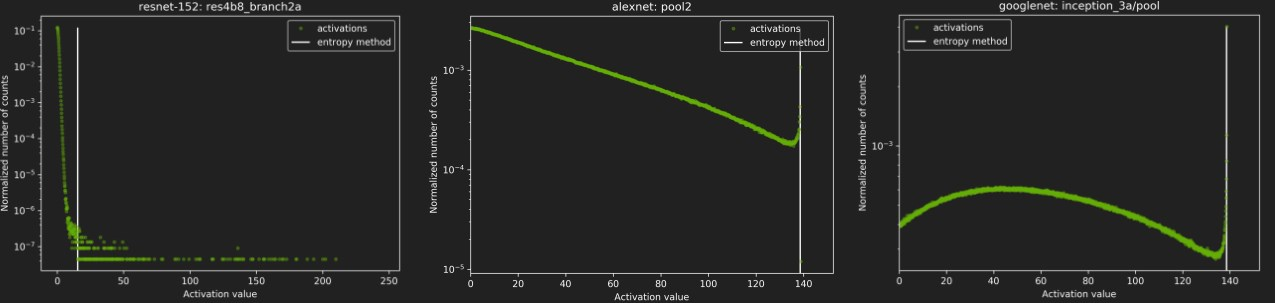### DP4A(Dot Product of 4 8-bits Accumulated to a 32-bit)

TensorRT 进行优化的方式是 DP4A (Dot Product of 4 8-bits Accumulated to a 32-bit)，如下图: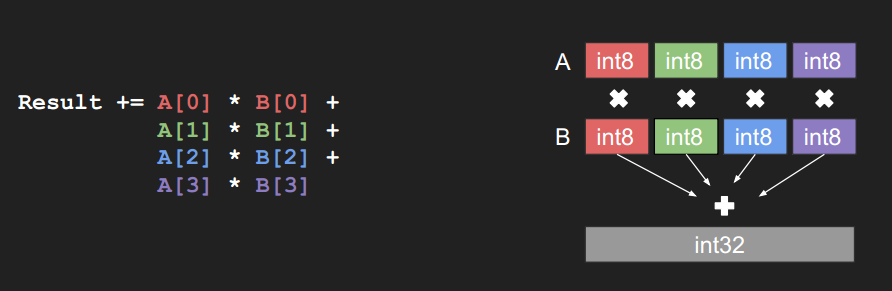// I8 input tensors: I8_input, I8_weights, INT8输入tensor
// I8 output tensors: I8_output， INT8输出tensor
// F32 bias (original bias from the F32 model),FP32的偏置
// F32 scaling factors: input_scale, output_scale, weights_scale[K], 这个是前面说的缩放因子sf
I32_gemm_out = I8_input * I8_weights // Compute INT8 GEMM (DP4A)，卷积计算，INT32输出
F32_gemm_out = (float)I32_gemm_out // Cast I32 GEMM output to F32 float，强制转换为FP32
//前面计算I8_input * I8_weights时，总的缩放系数为 input_scale * weights_scale[K]
//但是输出的缩放系数为output_scale，所以为了保证缩放程度匹配，要将F32_gemm_out乘以
//output_scale / (input_scale * weights_scale[ i ] )

// At this point we have F32_gemm_out which is scaled by ( input_scale * weights_scale[K] ),
// but to store the final result in int8 we need to have scale equal to "output_scale", so we have to rescale:
// (this multiplication is done in F32, *_gemm_out arrays are in NCHW format)
For i in 0, ... K-1:
rescaled_F32_gemm_out[ :, i, :, :] = F32_gemm_out[ :, i, :, :] * [ output_scale /(input_scale * weights_scale[ i ] ) ]

//将FP32精度的偏置 乘上缩放因子，加到前面的计算结果中
// Add bias, to perform addition we have to rescale original F32 bias so that it's scaled with "output_scale"
rescaled_F32_gemm_out _with_bias = rescaled_F32_gemm_out + output_scale * bias
//ReLU 激活
// Perform ReLU (in F32)
F32_result = ReLU(rescaled_F32_gemm_out _with_bias)
//重新转换为 INT8
// Convert to INT8 and save to global
I8_output = Saturate( Round_to_nearest_integer( F32_result ) )

### Typical workflow in TensorRT

• You will need:
• Model trained in FP32.
• Calibration dataset.
• TensorRT will:
• Run inference in FP32 on calibration dataset.
• Collect required statistics.
• Run calibration algorithm → optimal scaling factors.
• Quantize FP32 weights → INT8.
• Generate “CalibrationTable” and INT8 execution engine.

### Results - Accuracy & Performance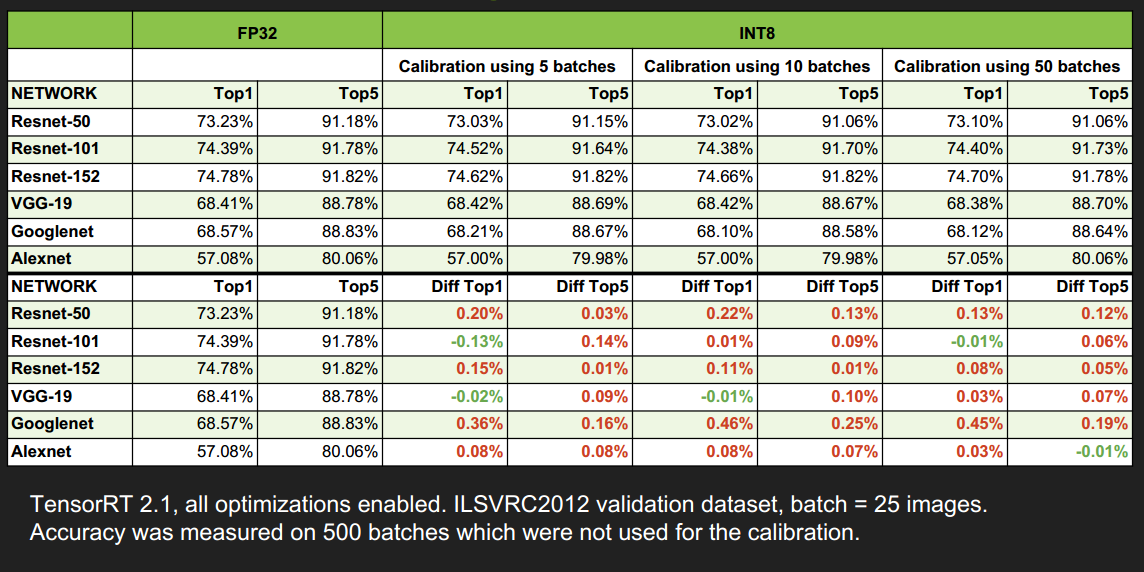速度提升还蛮多的，尤其是当 batch_size 大于1时，提升更明显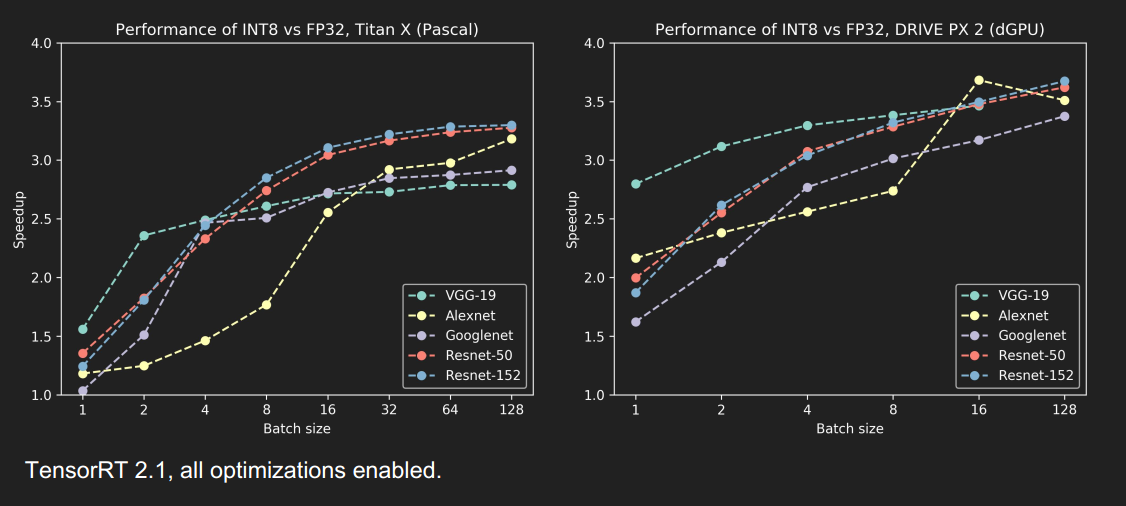TITAN X GPU优化效果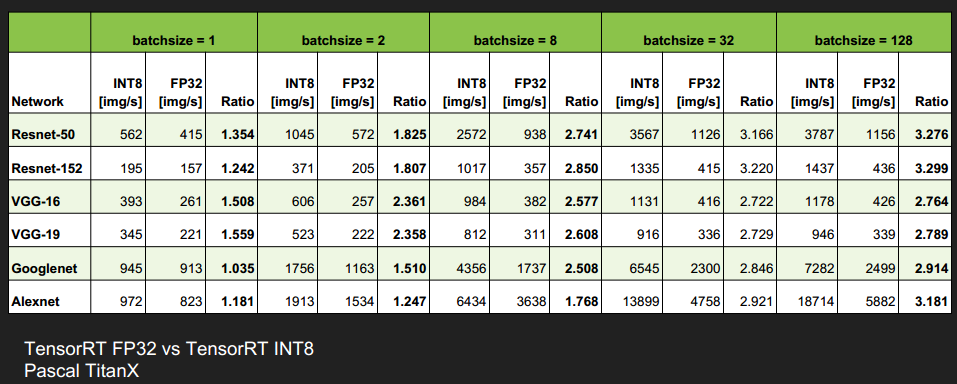DRIVE PX 2, dGPU 优化效果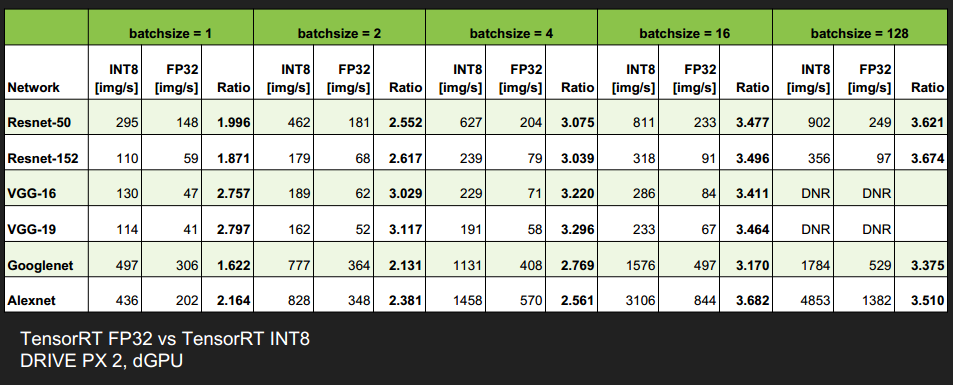### Open challenges / improvements

• Unsigned int8 for activations after ReLU. 无符号 INT8 的映射。
• RNNs → open research problem. TensorRT 3.0开始已经支持RNN了。
• Fine tuning of saturation thresholds. 对阈值 |T|的 微调方法。
• Expose API for accepting custom, user provided scale factors. 开放API，使用户可以自定义 换算系数（比例因子）

## Conclusion

• 介绍了一种自动化，无参数的 FP32 到 INT8 的转换方法；
• 对称的，不饱和的线性量化，会导致精度损失较大；
• 通过最小化 KL散度来选择 饱和量化中的 阈值 |T|;
• FP32完全可以降低为INT8推理，精度几乎持平，速度有很大提升。

## Reference

posted @ 2020-09-07 10:38  nanmi  阅读(4383)  评论(1编辑  收藏  举报